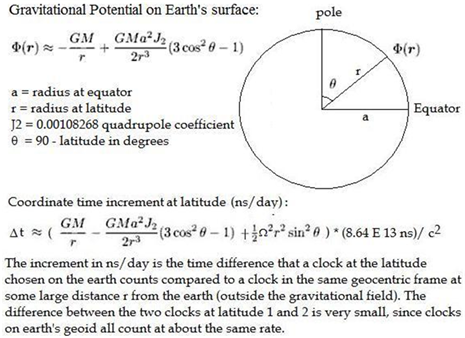Please fill or choose the data marked red and try submitting again.
 Gravitational Potential and Coordinate Time Increment on Earth's Oblate Geoid: Gravitational Potential: Grav. Calculation: Earth radius height ( h ) in air = 0 Grav. (1) Quadrupole (2) Centripetal (3) Earth radius (pole) degrees min at latitude above sea level in rock = 1 -GM/r (GMa^2J/2r^3)*(3cos^2q-1) (-W^2r^2sin^2q/2) Total: (1+2+3) F(r): (1+2) -GM/r^2 F(r)/r Total/r latitude 1 Earth radius average latitude 2 ratio: inv. ratio: Earth radius (equator) Kinematic Calculation:Clock Velocity E (m/s) trip duration (hrs) Rotv (m/s) ECIv (m/s) ECIv - Rotv (ns) Earth W average latitude 1 latitude 2 Earth Velocity rW Coordinate Time Increment at Latitude and height (h) (dt): cos(lat) sin(lat) pr2 =A Grav. Quadrupole Centripetal (-GM/r)/c^2 GMa^2J(3Cos^2q-1)/(2c^2r^3) (W*r*sinq)^2/2c^2 Total: a*a*cos(lat) b*b*sin(lat) Earth Mass a*cos(lat) b*sin(lat) ns in one day Coordinate Time Increment at Latitude and sea level (dt): cos(lat) sin(lat) G Grav. Quadrupole Centripetal (-GM/r)/c^2 GMa^2J(3Cos^2q-1)/(2c^2r^3) (W*r*sinq)^2/2c^2 Total: a*a*cos(lat) b*b*sin(lat) GM a*cos(lat) b*sin(lat) quadrupole coeff. J Hafele and Keating Experiment Calculation (n/s): c^2 Clock Velocity Dt Grav. Quadrupole Centripetal Total Grav.Dt Total in ns diff. (m) from ideal Geoid: latitude 1 m latitude 2 m diff. in ns Note: In the Hafale and Keating Simulation, the time differences shown for each moving clock (Lat.1 and Lat 2) are compared to a clock at sea level at latitude1 The sign of the velocities needs to be corrected for Latitude 2 when the ECIv exceeds the Rotv. Gravitational potential = mgh. Thus the ratios of the radius's = the inverse ratio of the g's at the different latitudes. This makes earth at sea level an equipotential surface.